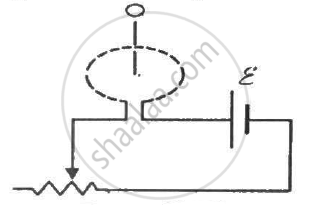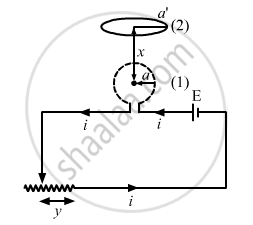Department of Pre-University Education, KarnatakaPUC Karnataka Science Class 12
Advertisement Remove all ads

# Figure Shows a Circular Coil Of N Turns and Radius A, Connected to a Battery of Emf εThrough a Rheostat. the Rheostat Has a Total Length L And Resistance R. the Resistance of the Coil Is R. - Physics

Sum

Figure shows a circular coil of N turns and radius a, connected to a battery of emf εthrough a rheostat. The rheostat has a total length L and resistance R. the resistance of the coil is r. A small circular loop of radius a' and resistance r' is placed coaxially with the coil. The centre of the loop is at a distance x from the centre of the coil. In the beginning, the sliding contact of the rheostat is at the left end and then onwards it is moved towards right at a constant speed v. Find the emf induced in the small circular loop at the instant (a) the contact begins to slide and (b) it has slid through half the length of the rheostat.Advertisement Remove all ads

#### SolutionThe magnetic field due to coil 1 at the centre of coil 2 is given by

$B = \frac{\mu_0 Ni a^2}{2 ( a^2 + x^2 )^{3/2}}$

The flux linked with coil 2 is given by

$\phi = B . A' = \frac{\mu_0 Ni a^2}{2 ( a^2 + x^2 )^{3/2}}\pi a '^2$

Now, let y be the distance of the sliding contact from its left end.

Given:-

$v = \frac{dy}{dt}$

Total resistance of the rheostat = R

When the distance of the sliding contact from the left end is y, the resistance of the rheostat (R') is given by

$R' = \frac{R}{L}y$

The current in the coil is the function of distance y travelled by the sliding contact of the rheostat. It is given by

$i = \frac{\epsilon}{\left( \frac{R}{L}y + r \right)}$

The magnitude of the emf induced can be calculated as:-

$e = \frac{d\phi}{dt} = \frac{\mu_0 N a^2 a '^2 \pi}{2 ( a^2 + x^2 )^{3/2}}\frac{di}{dt}$

$\Rightarrow e = \frac{\mu_0 N \pi a^2 a '^2}{2 ( a^2 + x^2 )^{3/2}}\frac{d}{dt}\frac{\epsilon}{\left( \frac{R}{L}y + r \right)}$

$\Rightarrow e = \frac{\mu_0 N \pi a^2 a '^2}{2 ( a^2 + x^2 )^{3/2}}\left[ \epsilon\frac{\left( - \frac{R}{L}v \right)}{\left( \frac{R}{L}y + r \right)^2} \right]$

(a) For y = L,

$e = \frac{\mu_0 N \pi a^2 a '^2 \epsilon Rv}{2L ( a^2 + x^2 )^{3/2} (R + r )^2}$

(b) For y = L/2,

$\frac{R}{L}y = \frac{R}{2}$

$\Rightarrow e = \frac{\mu_0 N \pi a^2 a '^2}{2L( a^2 + x^2 )^{3/2}}\frac{\epsilon Rv}{\left( \frac{R}{2} + r \right)^2}$

Concept: Induced Emf and Current
Is there an error in this question or solution?
Advertisement Remove all ads

#### APPEARS IN

HC Verma Class 11, Class 12 Concepts of Physics Vol. 2
Chapter 16 Electromagnetic Induction
Q 20 | Page 307
Advertisement Remove all ads

#### Video TutorialsVIEW ALL 

Advertisement Remove all ads
Share
Notifications

View all notifications

Forgot password?